# Knowledge Base

## Solution 34475: Finding the Area Between Curves on the TI-84 Plus C Silver Edition Graphing Calculator.

### How do I find the area between curves on the TI-84 Plus C Silver Edition graphing calculator?

To find the area between curves please see the below example:

Example: Find the area of the region bounded by:

f(x)=300x/(x2 + 625)
g(x)=3cos(.1x)
x=75

Solution:

1) Press [WINDOW] and set the values as below: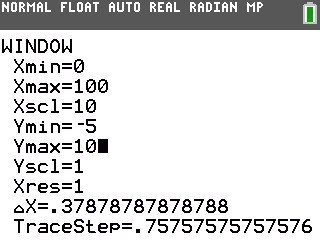2) Press [Y=] and make sure that no stat plots are highlighted.
3) Enter 300x/(x^2+625) in y1.
4) Enter 3cos(.1x) in y2.
5) Press [2nd][CALC] to select 5:intersect
6) Press [ENTER] for the first curve and [ENTER] for the second curve. To guess, move the cursor on the intersection of the graph on the left (The value of z at the intersection which is the lower limit of the integral is stored in Ans and X).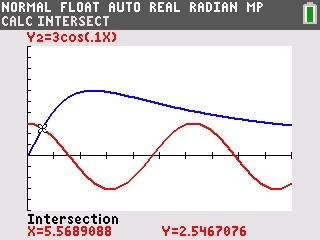7) Press [2nd][Quit] and press [2nd][DRAW] and use Shade( to see the area graphically. This will return to the home screen.
8) Press [VARS] and use the right arrow key to select Y-VARS.
9) Select 1:Function, press [ENTER] and select 2:Y2.
10) Press [,] [VARS] select Y-VARS and select 1: Function then select 1:Y1.
11) Press [,][2nd][ANS][,][)] [ENTER] (The screen should show Shade(Y2,Y1, Ans, 75)

The graph will look like the following: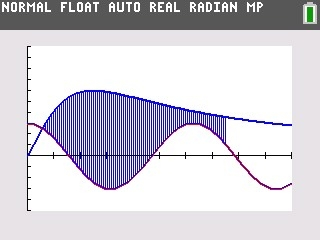12) Press [2nd][Quit] to return to the home screen. Enter the expression to evaluate the integral for the shaded region using the VAR menu and Ans as above.
13) Press [MATH] for fnInt( and enter Y1-Y2,x,Ans,75). If using TI-84 Plus in MATHPRINT mode, Ans is the lower bound, 75 is the upper bound, Y1-Y2 is the expression, and it needs to be in respects to X.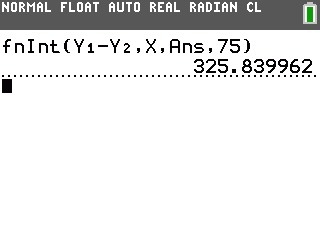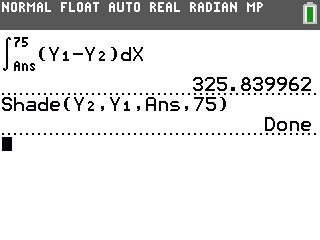The area is 325.84.

Please see the TI-84 Plus C Silver Edition guidebook for additional information.